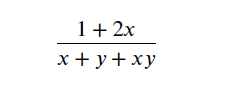# How to use Latex for Arithmetic operations

In this post, we’ll see how to use Latex to express Arithmetic operations.

A few points to remember:

– Inline formulas open and close with $or open with \ ( and close with \ ). – Displayed math environments open with \ [ and close with \ ]. – A math symbol is invoked by a command. For example, the command for ∞ is \infty and the command for → is \to. ## Latex for Arithmetic operations We type the arithmetic operations 𝑎 + 𝑏, 𝑎 − 𝑏, −𝑎, 𝑎∕𝑏, and 𝑎𝑏 in the natural way:$a + b$,$a – b$,$-a$,$a / b$, and$a b$(the spaces are typed only for readability). Related study: Display Roots with Latex ### Latex for multiplication operation If you wish to use ⋅ or × for multiplication, as in 𝑎⋅𝑏 or 𝑎×𝑏, use \cdot or \times, respectively. The formulas 𝑎⋅𝑏 and 𝑎×𝑏 are typed as$a \cdot b$and$a \times b$. ### Latex for division There is one form for division. 𝑎 ÷ 𝑏 is typed as follows:$a \div b$### Latex to display fraction using frac Let’s see how to handle Displayed fractions, such as the following:how to handle Displayed fractions with Latex. The fraction given is typed with \frac in the following way: \ [ \frac{1 + 2x}{x + y + xy} \ ] Here, \frac is the command, 1 + 2x and x + y + xy are the arguments. ### Displaying fraction using dfrac & tfrac You can use display-style fractions inline with \dfrac, and inline-style fractions in displayed math environments with \tfrac; for example, let’s take a fraction (3 + a2 ) / (4 + b). When this fraction needs to be shown with inline-style then it is to be typed as the following:$\dfrac{3 + a^{2}}{4 + b}$And, when fraction needs to be displayed in math environments then it’s to be typed as: [ \tfrac{3 + a^{2}}{4 + b} ] ## Subscripts and superscripts Subscripts are typed with _ and superscripts with ˆ (caret). Subscripts and superscripts should be enclosed in braces, that is, typed between { and }. To get 𝑎1, type$a_{1}$. Omitting the braces in the above example causes no harm, but to get 𝑎10, you must type$a_{10}$. Indeed,$a_10$is typeset as 𝑎10. Note: There is one symbol, the prime (’), that is automatically superscripted in a formula. To get 𝑓′(𝑥), just type$f’(x)\$.

One more example set with different types of subscripts and superscripts:

\ [
a_{1},\ a_{i_{1}},\ a^{2},\ a^{b^{c}},\ a^{i_{1}},\
a_{i} + 1,\ a_{i + 1},\ a_{1}^{2},\ a^{2}_{1}
\ ]

The above set typesets as